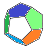MB Matheblog # 10 blog overviewprevious article    next article main pageindex website

 2021-05-06deutsche Version

Covering finite sets by subsets                    Comments are welcome

Introduction

The theory of partitions provides a well-known formula for the number of disjoint coverings of a finite set by a fixed number of non-empty subsets. In this paper, the number of all such (not necessarily disjoint) coverings is discussed, and a proof based on elementary combinatorics will be given.

The integer sequence OEIS A183109 $$(1, 1, 7, 1, 25, 265, 1, 79, 2161, 41503, ...)$$ in the OEISTM data base gives a start into this problem. This sequence describes the number of binary matrices with no zero rows or columns. For $$(n\times m)-$$matrices this number equals the number  $$u(n,m)$$ of coverings of a set containing  $$n$$  elements by  $$m$$  non-empty subsets (see section 2 below). The OEIS gives no proof. Riordan and Stein in  prove the result to be a special case of a much more general theorem which requires an elaborated deduction. A short proof will be presented in section 3.

Throughout this article,  $$M = \{m_1, ..., m_n\}$$  is a finite non-empty set.  $$(B_1,~...~,B_m)$$  is a  $$m-$$tuple of subsets of  $$M$$  and  $$(A_1,~...~,A_m)$$  is a  $$m-$$tuple of non-empty subsets of  $$M$$.

1.  Covering a set by disjoint subsets

If  $$(A_1,~...~,A_m)$$  is a disjoint covering of  $$M$$ ,  the set  $$\{A_1,~...~,A_m\}$$  is a  $$m-$$partition of  $$M$$. The number of  $$m-$$partitions of a set with  $$n$$  elements is given by the Stirling number of the second kind  $$S(n,m)$$ ,  cf. [2; 3.39]. $S(n,m) = \frac{1}{m!}\sum_{j=0}^m (-1)^{m-j} \binom{m}{j} j^n$ As  $$(A_1,~...~,A_m)$$  is an (ordered) $$m-$$tuple and the  $$A_i$$  are disjoint and thus pairwise different, there are  $$m!$$  arrangements of the  $$A_i$$ .  Therefore, the number  $$v(n,m)$$  of these tuples is $v(n,m) = m!\cdot S(n,m)$ Thus, the number of disjoint coverings  $$(A_1,~...~,A_m)$$  of  $$M$$  is $v(n,m) = \sum_{j=0}^m (-1)^{m-j} \binom{m}{j} j^n$ We quote this well-known result because the formula derived in section 3 for the non-disjoint case is of a very similar structure.

The OEIS data base contains  $$v(n,m)$$  as integer sequence OEIS A019538 $$(1, 1, 2, 1, 6, 6, 1, 14, 36, 24, 1, 30, 150, 240, 120, ...)$$.  $$v(n,m)$$  also represents the number of surjective mappings of a set with  $$n$$  elements onto a set with  $$m$$  elements. The sequence above is generated by the "triangle" with  $$v(n,m)$$  in the  $$n^{th}$$  row at the  $$m^{th}$$  position,  $$m = 1,~...~,n$$ ,  and read by rows. (The same principle applies to the sequence  $$u(n,m)$$  in the introduction which will be analyzed in section 3).

2.  Coverings and matrices

A  $$m-$$tuple  $$(B_1,~...~,B_m)$$  of subsets of  $$M = \{m_1,~...,~m_n\}$$  is conveniently described by a  $$(n\times m)-$$matrix  $$B$$  with entries  $$b_{ik} = 1$$  for  $$m_i \in B_k$$  and  $$b_{ik} = 0$$  else. So the  $$i^{th}$$  row of  $$B$$  indicates (by  $$b_{ik} = 1$$) which subsets  $$B_k$$  contain  $$m_i$$ ,  and the  $$k^{th}$$  column of  $$B$$  indicates which elements of  $$M$$  are also elements of  $$B_k$$ .  The same applies for  $$(A_1,~...~,A_m)$$  by substituting  $$A_k$$  for  $$B_k$$ .

$$(A_1,~...~,A_m)$$  covering  $$M$$  disjointly is equivalent to every row of  $$B$$  having exactly one entry  $$1$$  and every column having at least one entry  $$1$$ .

$$(A_1,~...~,A_m)$$  covering  $$M$$  arbitrarily as discussed in the next section is equivalent to every row and column of  $$B$$  having at least one entry  $$1$$ .

3.  Covering a set by arbitrary subsets

We derive the number  $$u(n,m)$$  of all  $$m-$$tuples  $$(A_1,~...~,A_m)$$  with  $$\bigcup A_k = M$$ : $\textbf{(1)}~~~\textbf{Theorem}~~~u(n,m) = \sum_{j=0}^m (-1)^{m-j} \binom{m}{j} (2^j-1)^n$ Proof.  Let  $$(B_1,~...~,B_m)$$  be a covering of  $$M$$ .  Recall that the subsets  $$B_k$$  may be empty. The  $$(n\times m)-$$matrix  $$B$$  is built as shown in section 2.

As  $$(B_1,~...~,B_m)$$  covers  $$M$$  each row of  $$B$$  must contain at least one entry  $$1$$ .  There are  $$2^m-1$$  different binary  $$m-$$vectors containing at least one  $$1$$ .  So we get: $\textbf{(2)}~~~\text{There are}~~(2^m-1)^n~~\text{different matrices}~~B.$ Each matrix  $$B$$  contains exactly  $$j$$  columns with at least one  $$1$$ ,  for some  $$j \in \{1,~...~,m\}$$ .  The corresponding  $$j$$  subsets  $$B_k$$  represent the covering of  $$M$$  and can be identified with  $$(A_1,~...~,A_j)$$  while the other  $$m-j$$  subsets are empty. If we call such a matrix  $$B$$  a  $$j-$$covering matrix we get $\textbf{(3)}~~~\text{There are}~~\binom{m}{j}\cdot u(n,j)~~~j\text{-covering}~~(n\times m)\text{-matrices.}$ Combining (2) and (3) yields $\textbf{(4)}~~~\sum_{j=1}^m \binom{m}{j} u(n,j) = (2^m-1)^n$ $$u(n,j)$$  in equation (4) are evaluated by the binomial inversion formula (5) for sequences  $$(a_j)$$  and  $$(c_j)$$ ;  cf. [2; 3.38(i)] : $\textbf{(5)}~~~\sum_{j=0}^m \binom{m}{j} a_j = c_m~~~~\Rightarrow~~~~a_m = \sum_{j=0}^m \binom{m}{j} (-1)^{m-j} c_j$ With  $$u(n,0) = 0~,~~a_j = u(n,j)$$  and  $$c_m = (2^m-1)^n$$  we get $u(n,m) = \sum_{j=0}^m \binom{m}{j} (-1)^{m-j} (2^j-1)^n$ This settles the claim of theorem (1).

4.  Remarks

(a)  $$u(n,m)$$  and  $$v(n,m)$$  are of similar build.

(b)  The number  $$m$$  of subsets can exceed the number  $$n$$  of elements. So why can  $$u(n,m)$$  be arranged in a "triangle"? This arrangement is used in OEIS and was described in the last paragraph of section 1. The answer proves the merit of the representation of a "$$m-$$covering" $$(A_1,~...~,A_m)$$  by the matrix  $$B$$  introduced in section 2.  $$B$$  is a binary  $$(n\times m)-$$matrix with no zero rows or columns. With regard to transposition, the number of these matrices equals the number of binary  $$(m\times n)-$$matrices with no zero rows or columns. This symmetry allows the restriction  $$m \le n$$.  -  An example: The number of  $$4-$$coverings of a set with  $$12$$  elements equals the number of  $$12-$$coverings of a set with  $$4$$  elements  $$(~u(12,4)=129,690,975,930,463~)$$.

(c)  Another way to obtain (1) would be an inclusion-exclusion proof.

References

 Riordan, J., Stein, P.R.:  Arrangements on chessboards, Journal of Combinatorial Theory, Series A Vol. 12(1), 1972, p. 72-80

 Aigner, M.:  Combinatorial Theory  (Springer, Berlin, 1997)